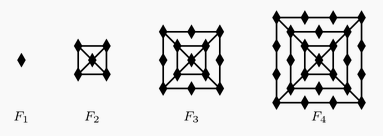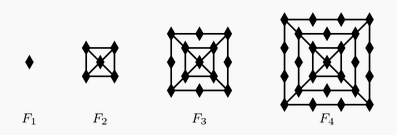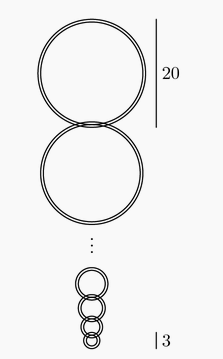###### back to index | new

The first two terms of a sequence are 10 and 20. If each term after the second term is the average of all of the preceding terms, what is the 2015th term?

The sequence $S_1, S_2, S_3, \cdots, S_{10}$ has the property that every term beginning with the third is the sum of the previous two. That is, $S_n = S_{n-2} + S_{n-1} \text{ for } n \ge 3.$ Suppose that $S_9 = 110$ and $S_7 = 42$. What is $S_4$?

Let $\{a_k\}_{k=1}^{2011}$ be the sequence of real numbers defined by $a_1=0.201,$ $a_2=(0.2011)^{a_1},$ $a_3=(0.20101)^{a_2},$ $a_4=(0.201011)^{a_3}$, and in general, $a_k=\begin{cases}(0.\underbrace{20101\cdots 0101}_{k+2\text{ digits}})^{a_{k-1}}\qquad\text{if }k\text{ is odd,}\\(0.\underbrace{20101\cdots 01011}_{k+2\text{ digits}})^{a_{k-1}}\qquad\text{if }k\text{ is even.}\end{cases}$ Rearranging the numbers in the sequence $\{a_k\}_{k=1}^{2011}$ in decreasing order produces a new sequence $\{b_k\}_{k=1}^{2011}$. What is the sum of all integers $k$, $1\le k \le 2011$, such that $a_k=b_k?$

Let $T_1$ be a triangle with sides $2011$, $2012$, and $2013$. For $n \geq 1$, if $T_n = \Delta ABC$ and $D, E$, and $F$ are the points of tangency of the incircle of $\Delta ABC$ to the sides $AB$, $BC$, and $AC$, respectively, then $T_{n+1}$ is a triangle with side lengths $AD, BE$, and $CF$, if it exists. What is the perimeter of the last triangle in the sequence $\left(T_n\right)$?

The figures $F_1$, $F_2$, $F_3$, and $F_4$ shown are the first in a sequence of figures. For $n\ge3$, $F_n$ is constructed from $F_{n - 1}$ by surrounding it with a square and placing one more diamond on each side of the new square than $F_{n - 1}$ had on each side of its outside square. For example, figure $F_3$ has $13$ diamonds. How many diamonds are there in figure $F_{20}$?The tower function of twos is defined recursively as follows: $T(1) = 2$ and $T(n + 1) = 2^{T(n)}$ for $n\ge1$. Let $A = (T(2009))^{T(2009)}$ and $B = (T(2009))^A$. What is the largest integer $k$ such that $\underbrace{\log_2\log_2\log_2\ldots\log_2B}_{k\text{ times}}$ is defined?

The first two terms of a sequence are $a_1 = 1$ and $a_2 = \frac {1}{\sqrt3}$. For $n\ge1$, $a_{n + 2} = \frac {a_n + a_{n + 1}}{1 - a_na_{n + 1}}.$ What is $|a_{2009}|$?

Let $a_1,a_2,\ldots$ be a sequence determined by the rule $a_n=a_{n-1}/2$ if $a_{n-1}$ is even and $a_n=3a_{n-1}+1$ if $a_{n-1}$ is odd. For how many positive integers $a_1 \le 2008$ is it true that $a_1$ is less than each of $a_2$, $a_3$, and $a_4$?

A sequence $(a_1,b_1)$, $(a_2,b_2)$, $(a_3,b_3)$, $\ldots$ of points in the coordinate plane satisfies $(a_{n + 1}, b_{n + 1}) = (\sqrt {3}a_n - b_n, \sqrt {3}b_n + a_n)$ for $n = 1,2,3,\ldots$. Suppose that $(a_{100},b_{100}) = (2,4)$. What is $a_1 + b_1$?

For each positive integer $n$, the mean of the first $n$ terms of a sequence is $n$. What is the $2008$th term of the sequence?

Given a finite sequence $S=(a_1,a_2,\ldots ,a_n)$ of $n$ real numbers, let $A(S)$ be the sequence $\left(\frac{a_1+a_2}{2},\frac{a_2+a_3}{2},\ldots ,\frac{a_{n-1}+a_n}{2}\right)$ of $n-1$ real numbers. Define $A^1(S)=A(S)$ and, for each integer $m$, $2\le m\le n-1$, define $A^m(S)=A(A^{m-1}(S))$. Suppose $x>0$, and let $S=(1,x,x^2,\ldots ,x^{100})$. If $A^{100}(S)=(1\/2^{50})$, then what is $x$?

A sequence $a_1,a_2,\dots$ of non-negative integers is defined by the rule $a_{n+2}=|a_{n+1}-a_n|$ for $n\geq 1$. If $a_1=999$, $a_2<999$ and $a_{2006}=1$, how many different values of $a_2$ are possible?

A sequence of numbers starts with $1$, $2$, and $3$. The fourth number of the sequence is the sum of the previous three numbers in the sequence: $1+2+3=6$. In the same way, every number after the fourth is the sum of the previous three numbers. What is the eighth number in the sequence?

For a particular sequence $a_1 = 3$, $a_2 = 5$ and $a_n = a_{n -1} - a_{n -2}$, for $n \ge 3$. What is the $2015^{th}$ term in this sequence?

Two non-decreasing sequences of nonnegative integers have different first terms. Each sequence has the property that each term beginning with the third is the sum of the previous two terms, and the seventh term of each sequence is $N$. What is the smallest possible value of $N$ ?

The figures $F_1$, $F_2$, $F_3$, and $F_4$ shown are the first in a sequence of figures. For $n\ge3$, $F_n$ is constructed from $F_{n - 1}$ by surrounding it with a square and placing one more diamond on each side of the new square than $F_{n - 1}$ had on each side of its outside square. For example, figure $F_3$ has $13$ diamonds. How many diamonds are there in figure $F_{20}$?Suppose that $(u_n)$ is a sequence of real numbers satifying $u_{n+2}=2u_{n+1}+u_n$, and that $u_3=9$ and $u_6=128$. What is $u_5$?

For each positive integer $n$, the mean of the first $n$ terms of a sequence is $n$. What is the $2008^{\text{th}}$ term of the sequence?

A number of linked rings, each 1 cm thick, are hanging on a peg. The top ring has an outside diameter of 20 cm. The outside diameter of each of the outer rings is 1 cm less than that of the ring above it. The bottom ring has an outside diameter of 3 cm. What is the distance, in cm, from the top of the top ring to the bottom of the bottom ring?Let $a_1 , a_2 , ...$ be a sequence for which$a_1=2$ , $a_2=3$, and $a_n=\frac{a_{n-1}}{a_{n-2}}$ for each positive integer $n \ge 3$. What is $a_{2006}$?

The first term of a sequence is $2005$. Each succeeding term is the sum of the cubes of the digits of the previous terms. What is the $2005^\text{th}$ term of the sequence?

In a sequence of positive integers, every term after the first two terms is the sum of the previous two terms of the sequence. The fifth term is 2012 so what is the maximum possible value of the first term?

Mike wrote a list of 6 positive integers on his paper. The first two are chosen randomly. Each of the remaining integers is the sum of the two previous integers. We are asked to find the ratio of the fifth integer to the sum of all 6 integers.

Let sequences {$a_n$} and {$b_n$} satisfy: $a_n=a_{n-1}\cos{\theta} - b_{n-1}\sin{\theta}$ and $b_n=a_{n-1}\sin{\theta}+b_{n-1}\cos{\theta}$. If $a_1=1$ and $b_1=\tan{\theta}$, where $\theta$ is a known real number, find the general formula for {$a_n$} and {$b_n$}.

If $x$ and $y$ are positive integer solutions to the equation $x^2 - 2y^2 = 1$, then $6\mid xy$.